# Experiment of The Month

## A lecture on laboratory realization of a Relativistic Clock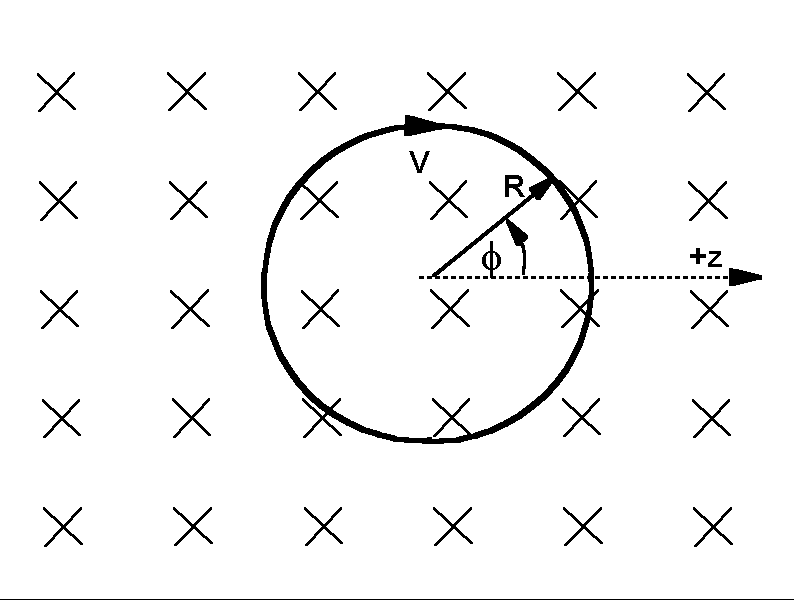In the fall semester of 1999, Dr. Dieter Forster https://phys.cst.temple.edu/dieter-forster.html spoke to the MU senior physics seminar. Although we have not done the experiments suggested, the talk was so interesting that a segment of it is transcribed for this months offering.

This segment describes a clock made from a charged particle orbiting in a magnetic field. (One trip around the orbit may be regarded as one "tick" of the clock.)

The figure shows an electron orbiting in a uniform magnetic field. The velocity of the electron is always in the plane of the picture, and the magnetic field is perpendicular to that plane, pointing into the "page."

The electron orbits with the familiar "cyclotron frequency," given by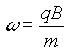, where q is the electric charge of the particle (-e in the case of the electron), m is its mass, and B is the strength of the applied magnetic field. The orbit radius, R is related to the orbit velocity, V, by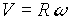We think of the circular orbit as occurring in the yz plane, with the magnetic field vector pointing in the +x direction. The centripetal force is provided by the magnetic "Lorentz force," in this case given by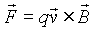.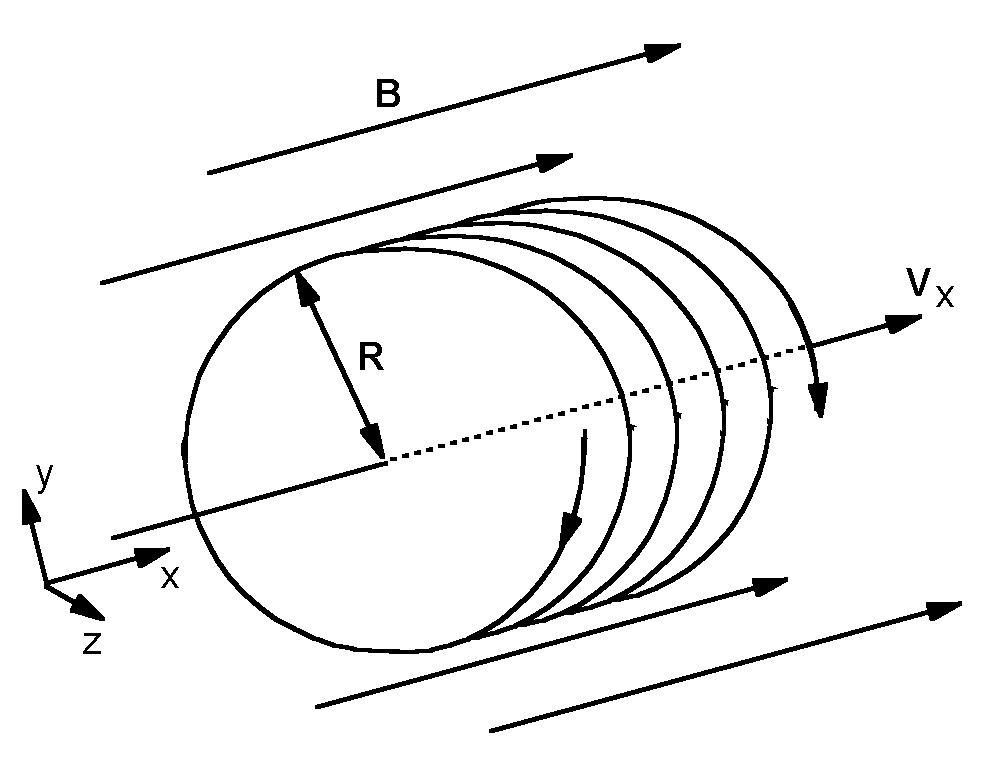If the particle has a component of velocity in the direction parallel to the magnetic field, that component has no effect on the "cyclotron orbit" in the xz plane. The symbolic reason is that the vector product ignores velocity components parallel to the magnetic field. The physical reason is that a charged particle moving parallel to a magnetic field experiences no magnetic force.

Dr. Forster used a mix of Einstein's relativity and classical physics to develop an experimental demonstration of "time dilation." In the experiment the clock is the orbiting charge. The period of the clock (the time to travel once around the orbit) changes when the x component of its velocity is changed. That is, the moving clock "runs slow," as predicted in a more general way by Einstein.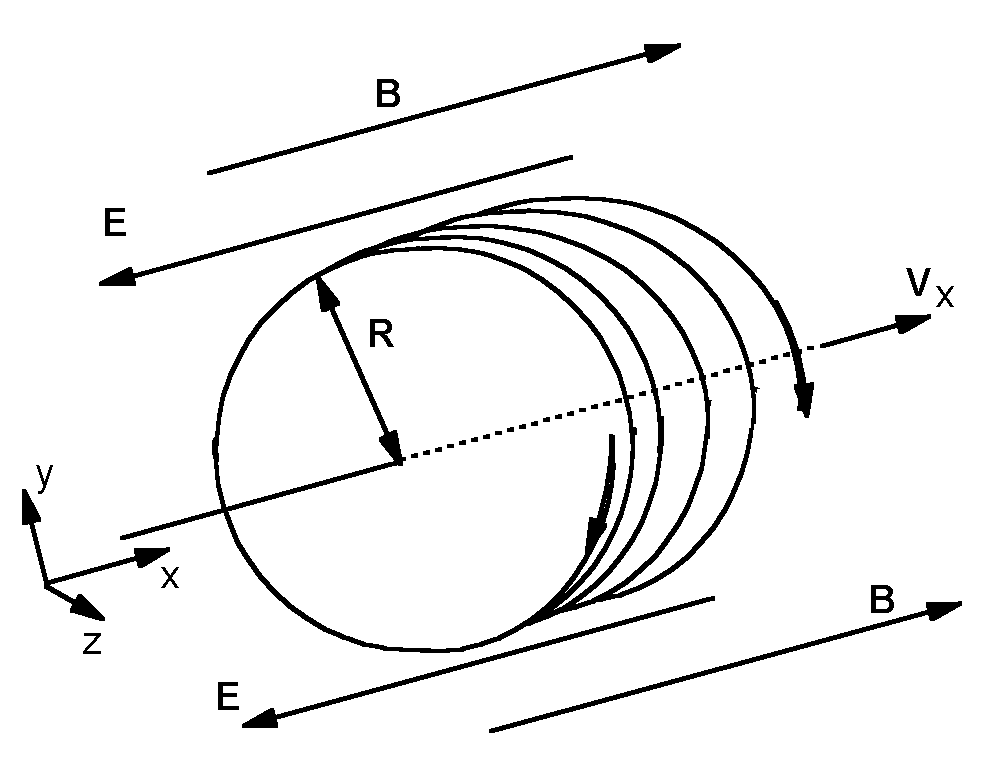To cause the change in the x component of velocity, an electric field, symbolized by E, is added to the experiment. The electric field accelerates the charge in the +x direction so that the x component of its velocity increases without any change in the motion in the yz plane. The effect of the acceleration will be to "increase the pitch" of the helix: As it speeds up, the charge will advance more in the x direction during the time it takes to complete one orbit.

In the figure, the electric field is represented by arrows. Because the charge in this example is negative, the electric field must point in the -x direction in order to create an acceleration in the +x direction.

The Lorentz force is now given by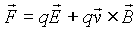. Conveniently, the magnetic component of the force acts only in the yz plane, and the electric component acts only in the x direction. Classically the effects of the two forces should be independent.

Dr. Forster used these classical forces along with a version of Newton's second law that is corrected to include Einstein's relativity. Recall that Newton wrote his second law (in modern notation) as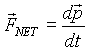, where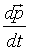is the rate of change of momentum.

Newton defined the momentum, p, as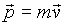The relativistic momentum is calculated in a slightly different way: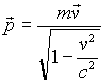, where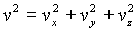is the magnitude of the velocity, and c is the speed of light. This difference is enough to force the famous speed limit, and also predicts strange behavior for moving clocks.

Dr. Forster proposed using the orbit period of the electron in a magnetic field as the clock "tick." He used the relativistic momentum and the (otherwise) ordinary Newton's second law with the ordinary electric and magnetic forces to predict the behavior of the clock .

The clock electron orbits in the yz plane, while the electric field accelerates it in the x direction. The problem breaks into two natural pieces: The motion in the x direction, and the motion in the plane perpendicular to the x direction (the yz plane).

Along the x direction, the rate of change of momentum is constant and equal only to the electric force (since the magnetic force is confined to the yz plane). This means that the momentum is simply proportional to time: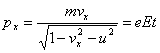.

In this equation, the y and z component of the velocity have been combined into the magnitude of the (tangential) velocity in the yz plane. Remember that the mass, m, is the rest mass. This can be solved for the x component of the velocity: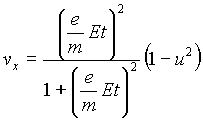.

For the yz plane, the magnitude of the momentum is constant. This is because the magnetic force acts perpendicular to the velocity and (thus) does no work. The magnetic force does not change the kinetic energy of the electron, and this means that the magnetic force does not change the magnitude of the electron momentum. The magnitude of the xy (or "perpendicular") momentum is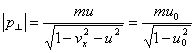where u0 is the initial value of the tangential velocity, and the initial value of the x component of the velocity is taken to be zero.

The momentum direction, however, does change. The rate of change of momentum is equal to the magnetic force. The z component of Newton's second law is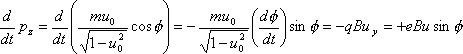where the z component of the vector product of u and B has been written out in terms of the y component of u.

Using the relation of u to the x component of v, this can be solved for the angular velocity in the yz plane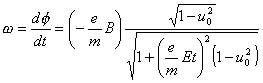.

The period of the orbit is given by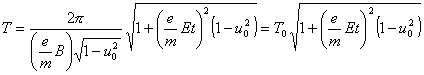,

and if this is written in terms of the x component of velocity, the clock period is (for small orbital velocity)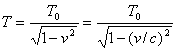.

This is the usual "time dilation," now appearing in a reasonably realistic moving clock. In the last part of the expression, we have converted back to mks units, so that the speed of light appears explicitly.